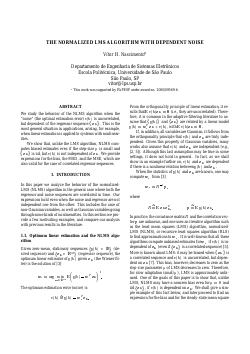##### The Normalized LMS Algorithm with Dependent Noise

###### Abstract
"We study the behavior of the NLMS algorithm when the “noise” (the optimal estimation error) v(k) is uncorrelated, but dependent of the regressor sequence {x_k}. This is the most general situation in applications, arising, for example, when linear estimation is applied to systems with nonlinearities. We show that, unlike the LMS algorithm, NLMS computes biased estimates even if the step-size mu is small and {x_k} is iid, but v(k) is not independent of x_k. We provide expressions for the bias, the MSD, and the MSE, which are also valid for the case of correlated regressor sequences."